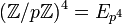# Group cohomology of elementary abelian group of prime-square order

(diff) ← Older revision | Latest revision (diff) | Newer revision → (diff)

Suppose$p$ is a prime number. We are interested in the elementary abelian group of prime-square order$E_{p^2} = (\mathbb{Z}/p\mathbb{Z})^2 = \mathbb{Z}/p\mathbb{Z} \oplus \mathbb{Z}/p\mathbb{Z}$.

## Homology groups

### Over the integers

$Z}/p\mathbb{Z} \oplus \mathbb{Z}/p\mathbb{Z};\mathbb{Z}) = \left\lbrace \begin{array}{rl} (\mathbb{Z}/p\mathbb{Z})^{(q + 3)/2} & \qquad q = 1,3,5,\dots \\ (\mathbb{Z}/p\mathbb{Z})^{q/2}, & q = 2,4,6,\dots \\ \mathbb{Z}, & \qquad q = 0 \\\end{array$

The first few homology groups are given below:$q$$0$$1$$2$$3$$4$$5$$H_q$$\mathbb{Z}$ $Z})^2 = E_{p^2$$\mathbb{Z}/p\mathbb{Z}$$(\mathbb{Z}/p\mathbb{Z})^3 = E_{p^3}$$(\mathbb{Z}/p\mathbb{Z})^2 = E_{p^2}$$(\mathbb{Z}/p\mathbb{Z})^4 = E_{p^4}$
rank of$H_q$ as an elementary abelian$p$-group -- 2 1 3 2 4

### Over an abelian group

The homology groups with coefficients in an abelian group$M$ are given as follows:$H_q(\mathbb{Z}/p\mathbb{Z} \oplus \mathbb{Z}/p\mathbb{Z};M) = \left\lbrace\begin{array}{rl} (M/pM)^{(q+3)/2} \oplus (\operatorname{Ann}_M(p))^{(q-1)/2}, & \qquad q = 1,3,5,\dots\\ (M/2M)^{q/2} \oplus (\operatorname{Ann}_M(p))^{(q+2)/2}, & \qquad q = 2,4,6,\dots \\ M, & \qquad q = 0 \\\end{array}\right.$

Here,$M/pM$ is the quotient of$M$ by$2M = \{ px \mid x \in M \}$ and$\operatorname{Ann}_M(p) = \{ x \in M \mid px = 0 \}$.

These homology groups can be computed in terms of the homology groups over integers using the universal coefficients theorem for group homology.

## Cohomology groups for trivial group action

### Over the integers

The cohomology groups with coefficients in the integers are given as below:$H^q(\mathbb{Z}/p\mathbb{Z} \oplus \mathbb{Z}/p\mathbb{Z};\mathbb{Z}) = \left\lbrace \begin{array}{rl} (\mathbb{Z}/p\mathbb{Z})^{(q-1)/2}, & q = 1,3,5,\dots \\ (\mathbb{Z}/p\mathbb{Z})^{(q+2)/2}, & q = 2,4,6,\dots \\ \mathbb{Z}, & q = 0 \\\end{array}\right.$

The first few cohomology groups are given below:$q$$\! 0$$\! 1$$\! 2$$\! 3$$\! 4$$\! 5$$H^q$$\mathbb{Z}$ 0$(\mathbb{Z}/p\mathbb{Z})^2 = E_{p^2}$$\mathbb{Z}/p\mathbb{Z}$$(\mathbb{Z}/p\mathbb{Z})^3 = E_{p^3}$$(\mathbb{Z}/p\mathbb{Z})^2 = E_{p^2}$
rank of$H^q$ as an elementary abelian$p$-group -- 0 2 1 3 2

### Over an abelian group

The cohomology groups with coefficients in an abelian group$M$ are given as follows:$H^q(\mathbb{Z}/p\mathbb{Z} \oplus \mathbb{Z}/p\mathbb{Z};M) = \left\lbrace \begin{array}{rl} (\operatorname{Ann}_M(p))^{(q+3)/2} \oplus (M/pM)^{(q-1)/2}, & q = 1,3,5,\dots \\ (\operatorname{Ann}_M(p))^{q/2} \oplus (M/pM)^{(q+2)/2}, & q = 2,4,6,\dots \\ M, & q = 0 \\\end{array}\right.$

Here,$M/pM$ is the quotient of$M$ by$pM = \{ px \mid x \in M \}$ and$\operatorname{Ann}_M(p) = \{ x \in M \mid px = 0 \}$.

These can be deduced from the homology groups with coefficients in the integers using the dual universal coefficients theorem for group cohomology.